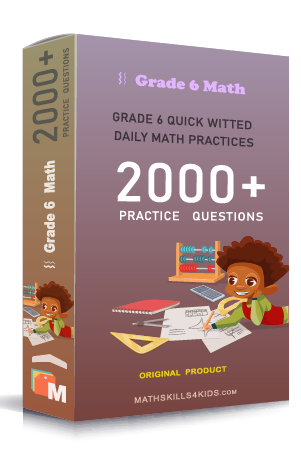# Grade 6 Money worksheets with solutions

Subject
Math

Resource Type
Worksheets, Printables, Homeschool

Format
PDF (11.0 MB | 14 pages → Exercises + Solutions)

• ### -15% OFF Over \$100

Promo Code: TOPLA

After Purchase

\$2.00

This package allows you to practice the following skills

Find the number of each type of coin

Add and subtract money amounts word problems

Multiply money by whole numbers and decimals

Multiply money word problems

Divide money amounts

Divide money amounts word problems

This product is a part of the Mega Pack
* Grade 6 quick-witted daily math practices *